Appendix A: Governing equations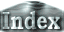A Boussinesq fluid is governed by the equations of heat, motion and continuity (e.g. Kimura, 1983):(14)(15)(16)

where, T is temperature,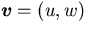is velocity,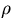is density, P is pressure,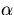is the thermal expansion coefficient,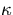is thermal diffusivity,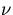is kinematic viscosity,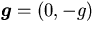is acceleration of gravity.

We introduce the stream function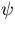as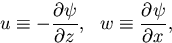(17)
Subsituting this into (14) and (15), we obtain(18)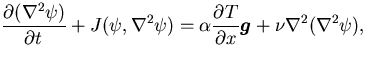(19)
where J(A,B) is the Jacobian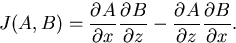(20)

The governing equations are non-dimensionalized using the thickness of the fluid layer b as the length scale, the thermal diffusion time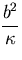as the time scale, and the temperature difference between the boundariesas the temperature scale, yielding,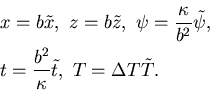Here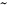denotes a non-dimensional variable. Equations (18) and (19) then become(21)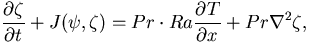(22)
where,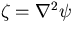is vorticity,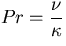is the Prandtl number, and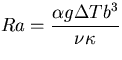is the Rayleigh number. The subscirptsdenoting a non-dimensional variable are omitted for simplicity.

A fixed temperature condition is specified at the top and the bottom boundaries: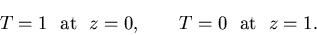(23)
The kinematic and dynamic boundary conditions are impermeable, and free-slip, such that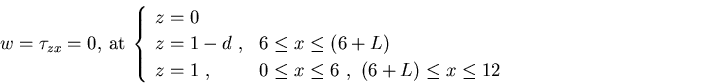(24)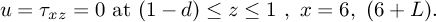(25)
Horizontal boundaries are periodic.

These kinematic and dynamic conditions are described by the voriticity and the stream function. The impermeable condition becomes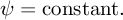(26)

Since the initial condtion is one of no motion and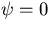, stream function always vanishes at the boundaries,(27)
Since w=0 at the horizontal plane of the boundaries, the free-slip condition becomesthat is,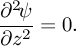(28)
Further, sinceat the horizontal plane of the boundaries, we have,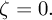(29)
By following the similar procedure for vertical plane of the boundaries, we obtain (29).

 Appendix A: Governing equations